# RC, RL and RLC Circuits

Published  June 15, 2018   0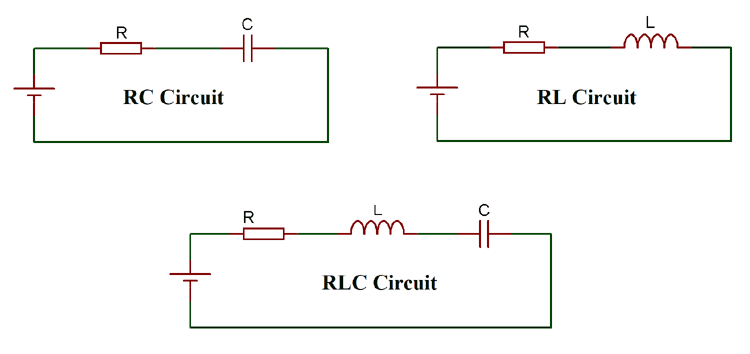The whole of electronics components can be slip into two broad categories, one being the Active components and the other as Passive components. The Passive components include the Resistor (R), Capacitor (C) and the Inductor (L). These are the three most used components in electronics circuit and you will find them in almost every application circuit. These three components together in different combinations will form the RC, RL and RLC circuits and they have many applications like from filtering circuits, Tube light chokes, multivibrators etc.. So in this tutorial we will learn the basic of these circuits, the theory behind them and how to use them in our circuits.

Before we jump into the main topics lets understand what an R, L and C does in a circuit.

Resistor: Resistors are denoted by the letter “R”. A resistor is an element that dissipates energy mostly in form of heat. It will have a Voltage drop across it which remains fixed for a fixed value of current flowing through it.

Capacitor: Capacitors are denoted by the letter “C”. A capacitor is an element which stores energy (temporarily) in form of electric field. Capacitor resists changes in voltage. There are many types of capacitors, out of which the ceramic capacitor and the electrolytic capacitors are mostly used. They charge in one direction and discharge in opposite direction

Inductor:  Inductors are denoted by the letter “L”. A Inductor is also similar to capacitor, it also stores energy but is stored in form of magnetic field. Inductors resist changes current. Inductors are normally a coil wound wire and is rarely used compared to the former two components.

When these Resistor, Capacitor and Inductors are put together we can form circuits like RC, RL and RLC circuit which exhibits time and frequency dependent responses that will e useful in many AC applications as mentioned already. A RC/RL/RLC circuit can be used as a filter, oscillator and much more it is not possible to cover every aspect in this tutorial, so we will learn the basic behaviour of them in this tutorial.

### Basic Principle of RC/RL and RLC circuits:

Before we start with each topic let us understand how a Resistor, Capacitor and an Inductor behave in an electronic circuit. For the purpose of understanding let us consider a simple circuit consisting of a capacitor and resistor in series with a power supply (5V). In this case when the power supply is connected to the RC pair, the voltage across the Resistor (Vr) increase to its maximum value while the voltage across the capacitor (Vc) stays at zero, then slowly the capacitor starts to build charge and thus the voltage across the resistor will decrease and the voltage across the capacitor will increase until the resistor voltage (Vr) has reached Zero and Capacitor voltage (Vc) has reached its maximum value. The circuit and the wave form can be seen in the GIF below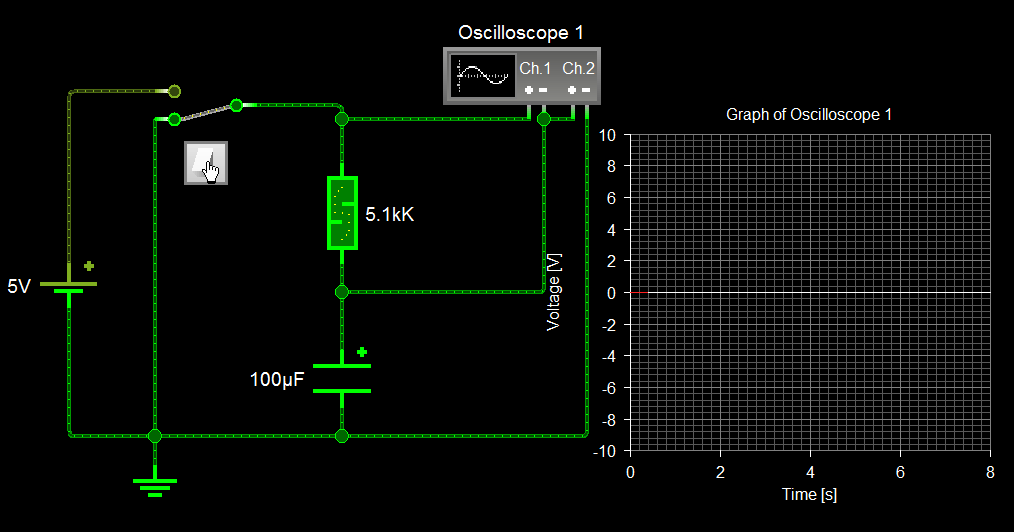Let us analyse the wave form in the above image to understand what is actual happening in the circuit is. A well illustrated waveform is show in the image below.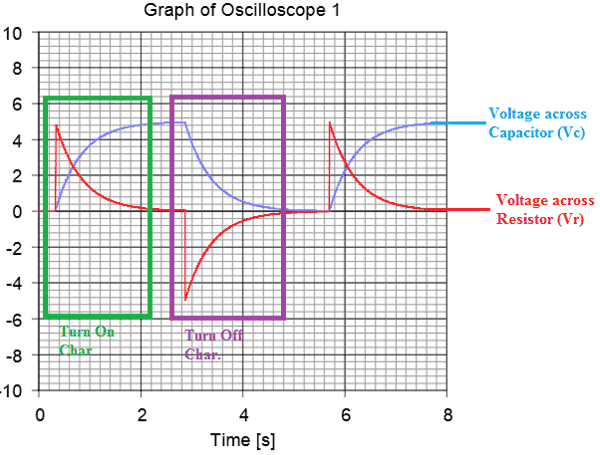When the switch is turned on the voltage across the resistor (red wave) reaches its maximum and the voltage across capacitor (blue wave) remains at zero. Then the capacitor charges up and Vr becomes zero and Vc becomes maximum. Similarly when the switch is turned off capacitor discharges and hence the negative voltage appears across the Resistor and as the capacitor discharges both the capacitor and resistor voltage becomes zero as shown above.

The same can be visualized for inductors as well.  Replace the capacitor with an inductor and the waveform will just be mirrored, that is the voltage across the resistor (Vr) will be zero when the switch is turned on since the whole voltage will appear across the Inductor (Vl). As the inductor charges up the voltage across (Vl) it will reach zero and the voltage across the resistor (Vr) will reach the maximum voltage.

### RC circuit:

The RC circuit (Resistor Capacitor Circuit) will consist of a Capacitor and a Resistor connected either in series or parallel to a voltage or current source. These types of circuits are also called as RC filters or RC networks since they are most commonly used in filtering applications. An RC circuit can be used to make some crude filters like low-pass, high-pass and Band-Pass filters. A first order RC circuit will consist of only one Resistor and one Capacitor and we will analyse the same in this tutorial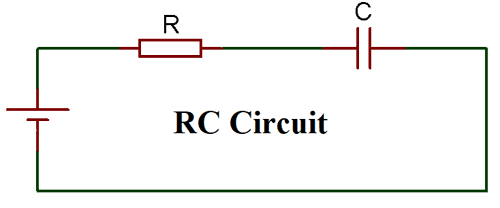To understand the RC circuit let us create a Basic circuit on proteus and connect the load across the scope to analyse how it behaves. The circuit along with the waveform is given below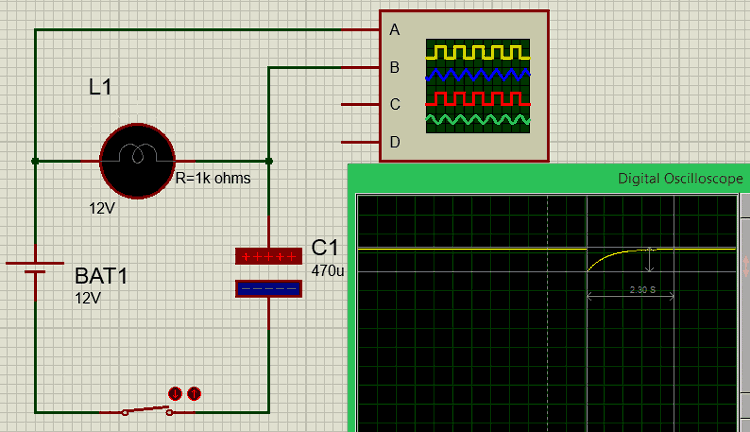We have connected a load (light bulb) of known resistance 1k Ohms in series with a capacitor of 470uF to form a RC circuit. The circuit is powered by a 12V battery and a switch is used to close and open the circuit. The waveform is measured across the load bulb and is shown in yellow colour on the image above.

Initially when the switch is open maximum voltage (12V) appears across the resistive light bulb load (Vr) and the voltage across the capacitor will be zero. When the switch is closed the voltage across the resistor will drop to zero and then as the capacitor charges the voltage will reach back to maximum as shown in the graph.

The time take for the capacitor to charge is given by the formulae T = 5Ƭ, where “Ƭ” represents tou (Time constant).

Let us calculate the time taken for our capacitor to charge up in the circuit.

```Ƭ = RC
= (1000 * (470*10^-6))
= 0.47 seconds

T = 5Ƭ
= (5 * 0.47)
T = 2.35 seconds.```

We have calculated that the time taken for the capacitor to charge up will be 2.35 seconds, the same can also be verified from the graph above. The time taken for Vr to reach from 0V to 12V is equal to the time taken for the capacitor to charge from 0V to maximum voltage. The graph is illustrated using the cursors in the below image.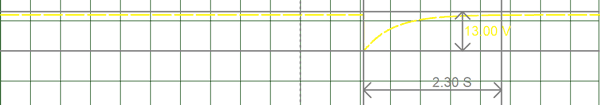Similarly we can also calculate the voltage across the capacitor at any given time and the current through the capacitor at any given time using the below formulas

```V(t) = VB (1 – e-t/RC)
I(t) =Io (1 – e-t/RC)```

Where, VB is the battery voltage and Io is the output current of the circuit. The value of t is the time (in seconds) at which the voltage or current value of the capacitor has to be calculated.

### RL circuit:

The RL Circuit (Resistor Inductor Circuit) will consist of an Inductor and a Resistor again connected either in series or parallel. A series RL circuit will be driven by voltage source and a parallel RL circuit will be driven by a current source. RL circuit are commonly used in as passive filters, a first order RL circuit with only one inductor and one capacitor is shown below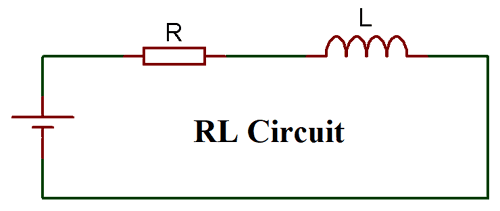Similarly in a RL circuit we have to replace the Capacitor with an Inductor. The Light bulb is assumed to act as a pure resistive load and the resistance of the bulb is set to a known value of 100 ohms.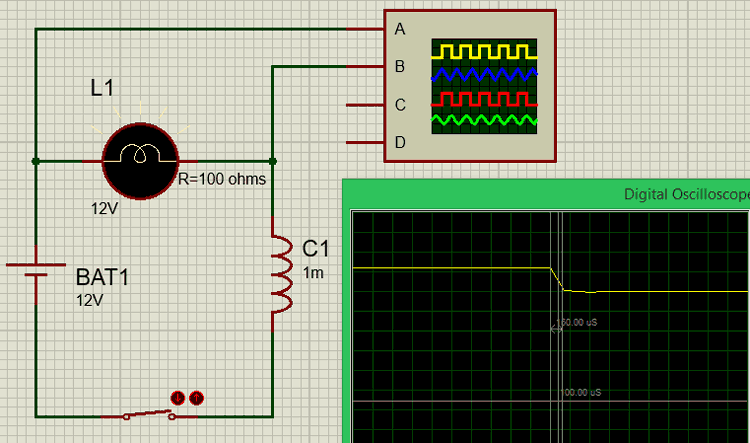When the circuit is open, the voltage across the resistive load will be maximum and when the switch is closed the voltage from the battery is shared between the inductor and the resistive load. The inductor charges up quickly and hence a sudden voltage drop will be experienced by the resistive load R.

The time taken for the inductor to charge up can be calculated using the formula T = 5Ƭ, where “Ƭ” represents tou (Time constant).

Let us calculate the time taken for our inductor to charge up in the circuit. Here we have used an inductor of value 1mH and the resistor of value 100 Ohms

```Ƭ = L/R
= (1 * 10^-3) / (100)
= 10^-5 seconds

T = 5Ƭ
= (5 * 10^-5)
= 50 * 10 ^-6
T = 50 u seconds.```

Similarly, we can also calculate the voltage across the Inductor at any given time and the current through the Inductor at any given time using the below formulas

```V(t) = VB (1 – e-tR/L)
I(t) =Io (1 – e-tR/L)```

Where, VB is the battery voltage and Io is the output current of the circuit. The value of t is the time (in seconds) at which the voltage or current value of the Inductor has to be calculated.

### RLC Circuit:

A RLC circuit as the name implies will consist of a Resistor, Capacitor and Inductor connected in series or parallel. The circuit forms an Oscillator circuit which is very commonly used in Radio receivers and televisions. It is also very commonly used as damper circuits in analog applications. The resonance property of a first order RLC circuit is discussed below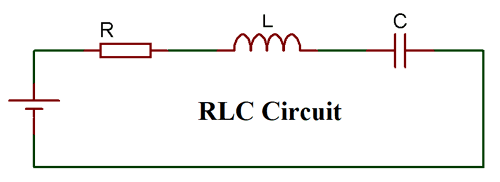The RLC circuit is also called as series resonance circuit, oscillating circuit or a tuned circuit. These circuit has the ability to provide a resonant frequency signal as shown in the below image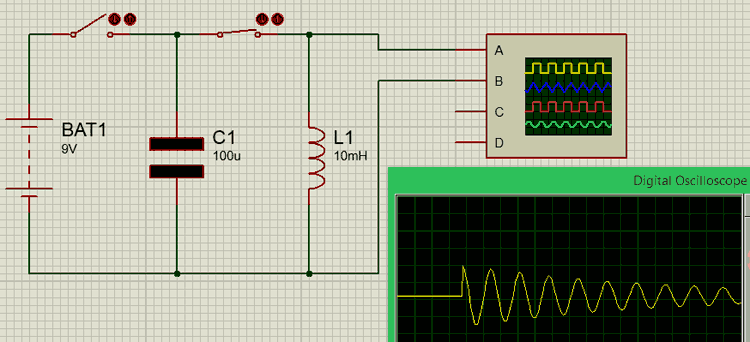Here we have a capacitor C1 of 100u and an Inductor L1 of 10mH connected tin series through a switch. Since the wire that is connecting the C and L will have some internal resistance, it is assumed that a small amount of resistance is present due to the wire.

Initially, we keep the switch 2 as open and close the switch 1 to charge up the capacitor from the battery source (9V). Then once the capacitor is charged the switch 1 is opened and then the switch 2 is closed.

As soon as the switch is closed the charge stored in the capacitor will move towards the inductor and charge it up. Once the capacitor is fully dis-charged, the inductor will start discharging back into the capacitor this way charges will flow to and fro between the inductor and the capacitor. But since there will be some loss in charges during this process total charge will gradually decrease until it reaches zero as shown in the graph above.

### Applications:

The Resistors, Inductors and Capacitors may be normal and simple components but when they are combined to gather to form circuits like RC/RL and RLC circuit they exhibit complex behaviour which makes it suitable for a wide range of application. Few of them are listed below

• Communication systems
• Signal Processing
• Voltage/Current magnification